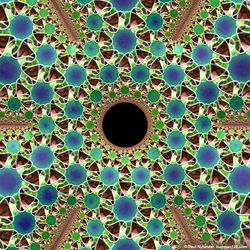top of page## rectified_icosahedral_honeycomb## Formula Mathematics Equation Mathematical Symbol Geometry Information Concept## MurlHoleSurfaceKnot-large## EGMO2017IrishTeam## honeycomb

Mandelbrot Set

The Mandelbrot set of complex numbers is defined by the recurrence relation zn+1=zn²+z0 (the set contains all complex numbers z0 that don't cause the the orbit of zn to diverge). This is a close-up of the fractal boundary of the set in the complex plane.

Dodecahedral Honeycomb

This is the order-4 dodecahedral honeycomb, with Schläfli symbol {5,3,4}, meaning it has four dodecahedra about each edge and eight around each vertex. One of many examples of honeycombs/tessellations of 3-D